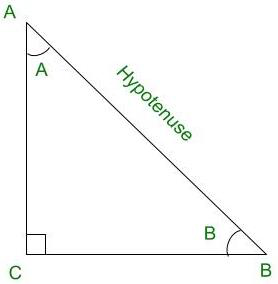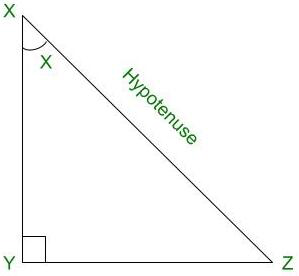Find sin2x, cos2x, and tan2x from the given information: cosec(x) = 6, and tan (x) < 0

• Last Updated : 12 Dec, 2021

Trigonometry is the relation between the angles and sides of a right-angled triangle. In a right angles triangle, there are 3 angles of which one angle is a right angle (90°) and other two angles are acute angles and there are 3 sides. The side opposite to the right angle is called Hypotenuse. There are 6 ratios between these sides based on the angle between them and they are called Trigonometric Ratios.

The 6 Trigonometric ratios are:

• Sine (sin)
• Cosine (cos)
• Tangent (tan)
• Cosecant (cosec)
• Secant (sec)
• Cotangent (cot)Right angled triangle ACB

Sine (sin):

Sine of an angle is defined by the ratio of lengths of sides which is opposite to the angle and the hypotenuse. For the above triangle, sin A = BC/AB

Cosine (cos):

Cosine of an angle is defined by the ratio of lengths of sides which is adjacent to the angle and the hypotenuse. For the above triangle, cos A = AC/AB

Tangent (tan):

Tangent of an angle is defined by the ratio of the length of sides which is opposite to the angle and the side which is adjacent to the angle. For the above triangle, tan A = BC/AC

Cosecant (cosec):

Cosecant of an angle is defined by the ratio of the length of the hypotenuse and the side opposite the angle. For the above triangle, cosec A = AB/BC

Secant (sec):

Secant of an angle is defined by the ratio of the length of the hypotenuse and the side and the side adjacent to the angle For the above triangle, sec A = AB/AC

Cotangent (cot):

Cotangent of an angle is defined by the ratio of length of sides which is adjacent to the angle and the side which is opposite to the angle. For the above triangle, cot A = AC/BC

Find sin2x, cos2x, and tan2x from the given information. csc (x) = 6, tan (x) < 0.

Solution:Right angled triangle XYZ

It is given that, cosecX = 6

We know that, sinX = 1/cosecX

⇒ sinX = 1/6                                                                ………………..( 1 )

⇒ sinX is positive

It is given that, tanX < 0

⇒ tanX is negative

Since, sinX is positive and tanX is negative

⇒ X lies in the 2nd Quadrant.

We know that, cos2X = 1 – sin2X

⇒ cos2X = 1 – (1/6)2

⇒ cos2X = 35 / 36

⇒ cosX = ± √35 / 6

But, since X lies in the 2nd Quadrant and Cosecant is negative in the 2nd Quadrant,

⇒ cosX = – √35 / 6                                                        ……………….(2)

sin(2X) = 2.sinX.cosX

From (1) and (2),

⇒ sin(2X) = 2.(1/6).(– √35 / 6)

⇒ sin(2X) = – √35/18                                               …………………(3)

cos(2X) = cos2X – sin2X

From (1) and (2),

⇒ cos(2X) = ( – √35 / 6)2 – (1 / 6)2

⇒ cos(2X) = 35 / 36 – 1 / 36

⇒ cos(2X) = 34 / 36

⇒ cos(2X) = 17 / 18                                                     …………………( 4 )

tan(2X) = sin(2X) / cos(2X)

From (3) and (4),

⇒ tan(2X) = (– √35 / 18) / (17 / 18)

⇒ tan(2X) = – √35 / 17

Therefore, the values of sin(2X), cos(2X) and tan(2X) are – √35 / 18, 17 / 18 and – √35 / 17 respectively.

Similar Questions

Question 1: Find sin(2X),cos(2X) and tan(2X) from given information: secX = 8, X lies in Quadrant IV

Solution:

It is given that, secX = 8

We know that, cosX = 1 / secX

⇒ cosX = 1 / 8                                                               ………………..( 1 )

We know that, sin2X = 1 – cos2X

⇒ sin2X = 1 – ( 1 / 8 )2

⇒ sin2X = 1 – 1/64

⇒ sin2X = 63 / 64

⇒ sinX = ± √63 / √64

⇒ sinX = ± 3√7 / 8

Since Sine( sin ) is negative in Quadrant IV,

⇒ sinX = – 3√7 / 8                                                        ……………….( 2 )

sin( 2X ) = 2.sinX.cosX

From (1 ) and ( 2 ),

⇒ sin( 2X ) = 2.( – 3√7 / 8).(1 / 8)

⇒ sin( 2X ) = – 3√7 / 32                                             ………………..( 3 )

cos( 2X ) = cos2X – sin2X

From (1 ) and ( 2 ),

⇒ cos( 2X ) = (1 / 8)2 – (– 3√7 / 8)2

⇒ cos( 2X ) = (1 / 64) – (63 / 64)

⇒ cos( 2X ) = – 62 / 64

⇒ cos( 2X ) = – 31 / 32                                               ………………..( 4 )

tan( 2X ) = sin( 2X ) / cos( 2X )

From (3 ) and ( 4 ),

⇒ tan( 2X ) = (– 3√7 / 32) / ( – 31 / 32)

⇒ tan( 2X ) =  3√7 / 31

Therefore, the values of sin( 2X ), cos( 2X ) and tan( 2X ) are – 3√7 / 32, – 31 / 32 and 3√7 / 31 respectively.

Question 2: Find sin(2X), cos(2X) and tan(2X) from given information: sinX = 3/5, X lies in Quadrant I

Solution:

It is given that, sinX = 3/5                                        ……………….( 1 )

We know that, cos2X = 1 – sin2X

⇒ cos2X = 1 – ( 3 / 5 )2

⇒ cos2X = 16 / 25

⇒ cosX = ± 4 / 5

Since X lies in the 1st Quadrant and Cosecant is positive in the 1st Quadrant,

⇒ cosX = 4 / 5                                                              ……………….( 2 )

sin( 2X ) = 2.sinX.cosX

From (1 ) and ( 2 ),

⇒ sin( 2X ) = 2.( 3/5 ).( 4/5)

⇒ sin( 2X ) = 24 / 25                                            ………………..( 3 )

cos( 2X ) = cos2X – sin2X

From (1 ) and ( 2 ),

⇒ cos( 2X ) = ( 4/5 )2 – ( 3/5 )2

⇒ cos( 2X ) = 16/25 – 9/25

⇒ cos( 2X ) = 7/25                                             …………………..( 4 )

tan( 2X ) = sin( 2X ) / cos(2X)

From (3 ) and ( 4 ),

⇒ tan( 2X ) = ( 24/25 ) / (7/25)

⇒ tan( 2X ) =  24/7

Therefore, the values of sin(2X), cos(2X) and tan(2X) are 24 / 25, 7/25 and 24/7 respectively.

Question 3: Find sin(2X),cos(2X) and tan(2X) from given information: cosX = 15/17, X lies in Quadrant IV

Solution:

It is given that, cosX = 15/17                                        ……………….(1)

We know that, sin2X = 1 – cos2X

⇒ sin2X = 1 – ( 15/17 )2

⇒ sin2X = 1 – 225 / 289

⇒ sin2X = 64 / 289

⇒ sinX = ± 8 / 17

Since X lies in the 1st Quadrant and Sine( sin ) is positive in the 4th Quadrant,

⇒ sinX = – 8/17                                                             ……………….(2)

sin( 2X ) = 2.sinX.cosX

From (1) and (2),

⇒ sin( 2X ) = 2.( – 8/17 ).(15/17)

⇒ sin(2X) = – 240/289                                            ………………..( 3 )

cos(2X) = cos2X – sin2X

From (1) and (2),

⇒ cos(2X) = (15/17)2 – (– 8/17)2

⇒ cos(2X) = 225/289 – 64/289

⇒ cos(2X) = 161/289                                            …………………..( 4 )

tan(2X) = sin(2X) / cos(2X)

From (3) and (4),

⇒ tan(2X) = (– 240/289) / (161/289)

⇒ tan(2X) = – 240/289

Therefore, the values of sin(2X), cos(2X) and tan(2X) are – 240/289, 161/289 and – 240/289 respectively.

My Personal Notes arrow_drop_up